Author: Oscar Cronquist Article last updated on February 17, 2023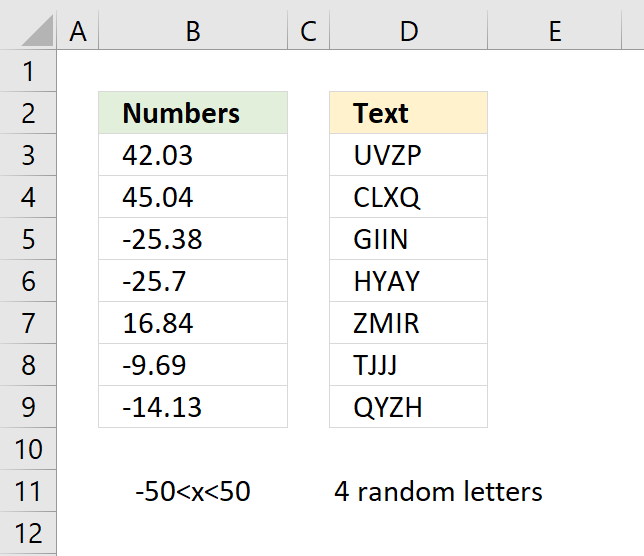Microsoft Excel has three useful functions for generating random numbers, the RAND, RANDBETWEEN, and the RANDARRAY functions.

The RAND function returns a random decimal number equal to or larger than 0 (zero) and smaller than 1. Example: 0.600842025092928

The RANDARRAY function calculates an array of random numbers. It allows you to choose integers or decimal values, how many rows and columns to fill and the number range.

This is a new function available for Excel 365 subscribers in the monthly channel, it automatically spills values across rows and columns without the need to enter it as an array formula. This is called spilled array behavior and is a new feature in Excel.

RANDBETWEEN function returns random integers in a range you specify. The function has two arguments: bottom and top. An integer is a whole number (contrary a fractional number) that can be positive, negative, or zero.

RANDBETWEEN(bottom, top)

Bottom is the lowest number in the range of numbers you want to return. Top is the highest number in the range.

Example, RANDBETWEEN(1,10) generates random numbers between 1 to 10.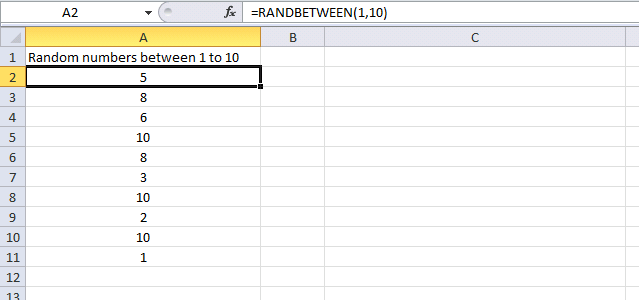Formula in cell range A2:A11:

=RANDBETWEEN(1,10)

As shown above, there are duplicates. If you don't want duplicates, read this post: How to create a list of random unique numbers

### Generate numbers with a decimal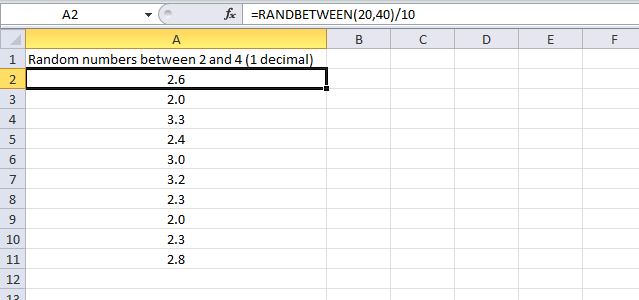In this example, the RANDBETWEEN function generates random numbers between 2 and 4 with a decimal.

Formula in cell range A2:A11:

=RANDBETWEEN(20,40)/10

Since it actually can't generate numbers with decimals the function generates values between 20 to 40. Divide the output with 10 and the range becomes 2.0 to 4.0.

### Generate negative numbers with two decimals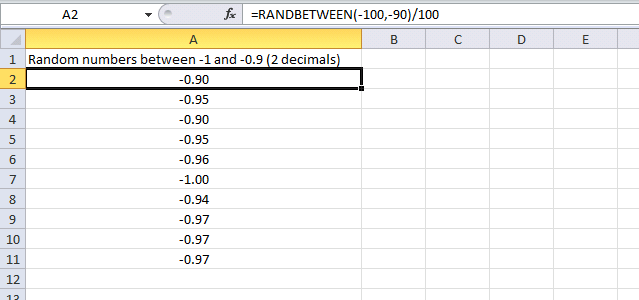In this example, the RANDBETWEEN function generates random numbers between -1 and -0.9 with two decimals.

Formula in cell range A2:A11:

=RANDBETWEEN(-100,-90)/100

The function generates numbers between -100 and -90. The returned number is then divided by 100. The range becomes -1.00 to -0.90.

### Generate negative numbers with three decimals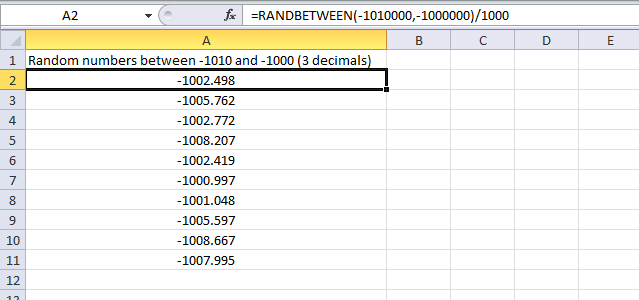Here, the RANDBETWEEN function generates random negative numbers between -1010.000 and -1000.000

Formula in cell range A2:A11:

=RANDBETWEEN(-1010000,-1000000)/1000

The function generates numbers between -1010000 and -1000000. The returned number is then divided by 1000. The range becomes -1010.000 to -1000.000

### Generate random names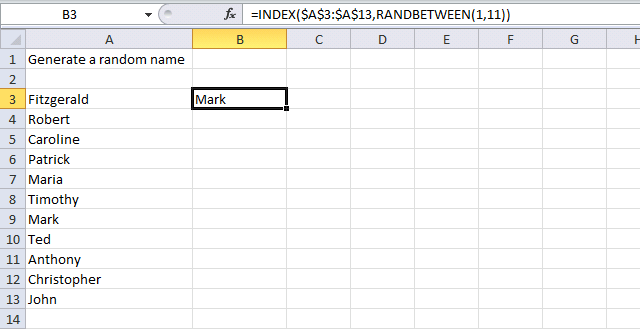The formula in cell B3 returns random names from cell range A3:A13.

Formula:

=INDEX(\$A\$3:\$A\$13,RANDBETWEEN(1,11))

### Explaining formula in cell B3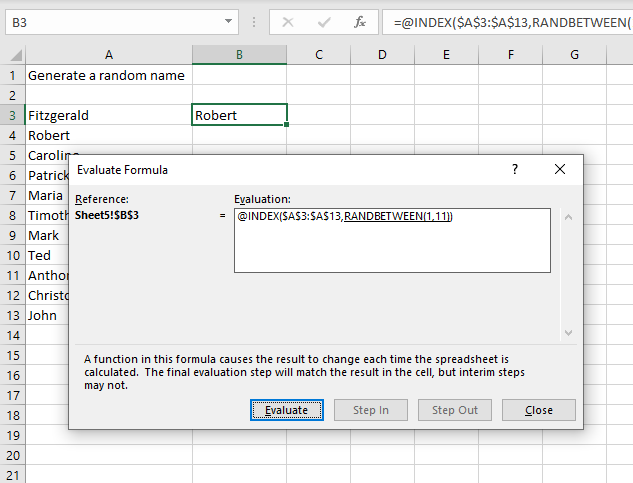I recommend that you use Excel's built-in tool for examining formulas, it is a great feature that allows you to see the calculations steps at a pace you are comfortable with.

Go to tab "Formulas" on the ribbon. Press with mouse on "Evaluate Formula" button to open the "Evaluate Formula" dialog box.

Press with left mouse button on "Evaluate" button to move the calculation to the next step, continue press with left mouse button oning the "Evaluate" button until all steps have been shown. Press with left mouse button on the "Close" to dismiss the dialog box.

#### Step 1 - Generate random value between 1 and 11

RANDBETWEEN(1,11) returns a number between 1 to 11

#### Step 2 - Return a value of the cell at the intersection of a particular row and column

The INDEX function allows you to return a value from a given range.

INDEX(array, row_num, [column_num])

INDEX(\$A\$3:\$A\$13, random_value)

The given range is \$A\$3:\$A\$13 and there are 11 cells in that range. Therefore, we need to return a random value between 1 and 11.

RANDBETWEEN(1, 11) returns a number between 1 to 11. The INDEX function then returns a random value from cell range \$A\$3:\$A\$13 in cell B3.

You can use the formula in the following post to return unique distinct names: How to create a list of random unique numbers

### Generate a random list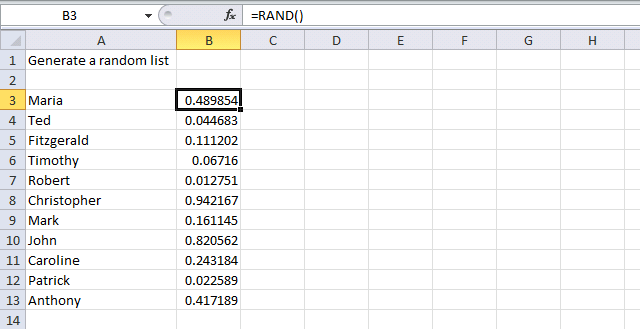The following steps show you how to randomly shuffle a list. The image above shows names in cell range A3:A13.

1. Select cell range B3:B13
2. Type =RAND()
3. Press and hold CTRL + Enter. This will enter the formula in all cells at once, no need to copy the formula and paste to cells below.
4. Select cell range A3:B13
5. Press with right mouse button on on the selected cell range.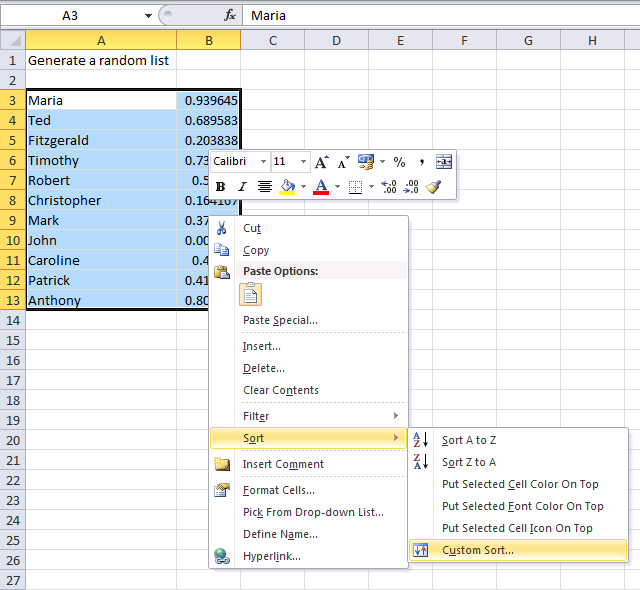6. Press with left mouse button on Sort.
7. Press with left mouse button on "Custom Sort..." and a dialog box appears.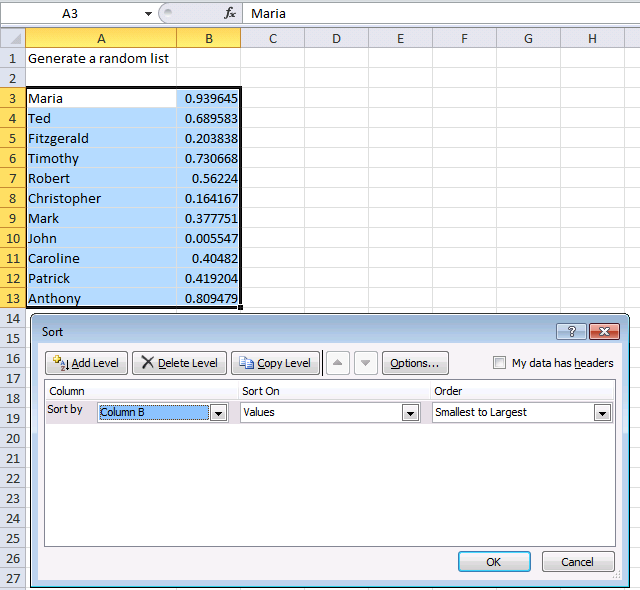8. Sort by: Column B
9. Order: Smallest to Largest
10. Press with left mouse button on OK button.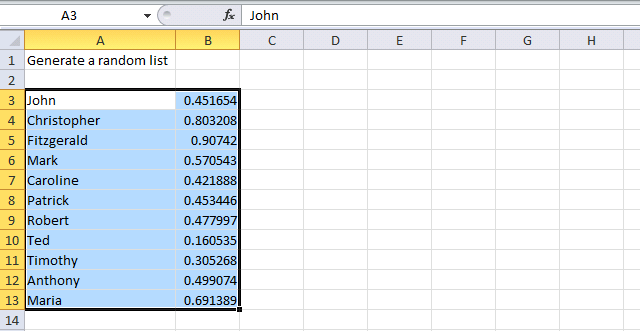The image above shows the list sorted in a random order.

### Create random text strings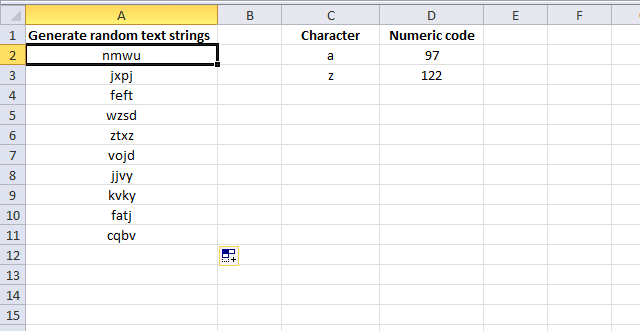The image above demonstrates a formula in cell range A2:A11 that returns four random characters concatenated into one text string. The characters are lower letters between a and z.

Formula:

=CHAR(RANDBETWEEN(97,122))& CHAR(RANDBETWEEN(97,122))& CHAR(RANDBETWEEN(97,122))& CHAR(RANDBETWEEN(97,122))

### Explaining formula in cell A2

#### Step 1 - Return a random number between 97 and 122

So why return a number between 97 and 122? All characters have a numeric code and the CODE function lets you convert a character to a numeric code.

For example, a is 97 and z is 122. By generating random numbers between 97 and 122 we can create characters from a to z.

RANDBETWEEN(97,122)

#### Step 2 - Return a character specified by a code number

The CHAR function converts a number to the corresponding character. This is determined by your computer's character set.

CHAR(RANDBETWEEN(97,122))

returns a random character from a to z.

#### Step 3 - Combine four characters

The ampersand operator & combines different functions to a text string. The are four instances of the formula above, the ampersand concatenates the output from each and returns a text string containing four lower letters.

CHAR(RANDBETWEEN(97,122))& CHAR(RANDBETWEEN(97,122))& CHAR(RANDBETWEEN(97,122))& CHAR(RANDBETWEEN(97,122))